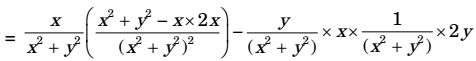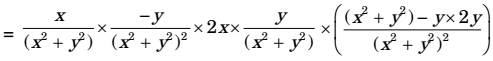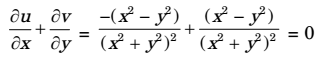## Fluid Mechanics and Hydraulic Machinery Miscellaneous

#### Fluid Mechanics and Hydraulic Machinery

1. For a steady flow, the velocity field is V = (–x² + 3y) i + (2xy)j. The magnitude of the acceleration of a particle at (1, - 1) is

1. NA

##### Correct Option: C

NA

1. For a two-dimensional flow, the velocity field is
 u = x i + y j x² + y² x² + y²

where i and j are the basis vectors in the x – y Cartesian coordinate system. Identify the CORRECT statements from below.
1. The flow is incompressible
3. y-component of acceleration,
 ay = - y (x² + y²)²

4. x-component of acceleration,
 ax = -(x + y) (x² + y²)²

1.  axu δu + v δu δx δy= x(x² + y² -2x²)-2xy² = - x3 - xy² (x² + y²)(x² + y²) (x² + y²)

 ∴ ax = x (x² + y²)²

 ay = u δv + v δv δx δy= -x² + yx² - y3 = - y (x² + y²)3 (x² + y²)²

The velocity components are not functions of time, so flow is steady according to continuity equation,Since it satisfies the above continuity equation for 2D and incompressible flow.
∴ The flow is incompressible.

##### Correct Option: B

 axu δu + v δu δx δy= x(x² + y² -2x²)-2xy² = - x3 - xy² (x² + y²)(x² + y²) (x² + y²)

 ∴ ax = x (x² + y²)²

 ay = u δv + v δv δx δy= -x² + yx² - y3 = - y (x² + y²)3 (x² + y²)²

The velocity components are not functions of time, so flow is steady according to continuity equation,Since it satisfies the above continuity equation for 2D and incompressible flow.
∴ The flow is incompressible.

1. For a certain two-dimensional incompressible flow, velocity field is given by 2xyi – y² j. The streamlines for this flow are given by the family of curves

1. v = 2xy i – y²j

 u = δx , v = - δx δy δx

 2xy = δx = 2 δy

2xyδy = δψ
on integrating
ψ = xy² + f(x)
= y1 + f'(x)
f'(x) = 0
⇒ f(x)= constant
so ψ= xy² + constant

##### Correct Option: B

v = 2xy i – y²j

 u = δx , v = - δx δy δx

 2xy = δx = 2 δy

2xyδy = δψ
on integrating
ψ = xy² + f(x)
= y1 + f'(x)
f'(x) = 0
⇒ f(x)= constant
so ψ= xy² + constant

1. The volumetric flow rate (per unit depth) between two streamlines having stream function ψ1 and ψ2 is

1. NA

##### Correct Option: D

NA

1. The velocity field of an incompressible flow is given by V = (a1x + a2y + a3 z)i + (b1x + b2y + b3 z)j + (c1x + c2y + c3 z)k, where a1 = 2 and c3 = –4. The value of b2 is _________.

1.  δu + δv + δw = 0 δx δy δz

a1 + b2 + c3 = 0
2 – 4 + b2 = 0
b2 = 2

##### Correct Option: A

 δu + δv + δw = 0 δx δy δz

a1 + b2 + c3 = 0
2 – 4 + b2 = 0
b2 = 2